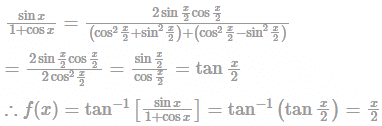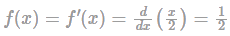If f(x) = tan−1⁡[sin⁡x/1+cos⁡x], then what i...

### Related TestIf f(x) = tan−1⁡[sin⁡x/1+cos⁡x], then what is the first derivative of f(x)?Kunaal SatijaLet us express (sinx/1+cosx) in terms of tan⁡x.And, the first derivative ofHence, the correct answer is 1/2.View courses related to this question Explore CAT courses
 Explore CAT coursesView courses related to this question1 Crore+ students have signed up on EduRev. Have you?

### Similar CAT Doubts

Question Description
If f(x) = tan−1⁡[sin⁡x/1+cos⁡x], then what is the first derivative of f(x)?Correct answer is '1/2'. Can you explain this answer? for CAT 2023 is part of CAT preparation. The Question and answers have been prepared according to the CAT exam syllabus. Information about If f(x) = tan−1⁡[sin⁡x/1+cos⁡x], then what is the first derivative of f(x)?Correct answer is '1/2'. Can you explain this answer? covers all topics & solutions for CAT 2023 Exam. Find important definitions, questions, meanings, examples, exercises and tests below for If f(x) = tan−1⁡[sin⁡x/1+cos⁡x], then what is the first derivative of f(x)?Correct answer is '1/2'. Can you explain this answer?.
Solutions for If f(x) = tan−1⁡[sin⁡x/1+cos⁡x], then what is the first derivative of f(x)?Correct answer is '1/2'. Can you explain this answer? in English & in Hindi are available as part of our courses for CAT. Download more important topics, notes, lectures and mock test series for CAT Exam by signing up for free.
Here you can find the meaning of If f(x) = tan−1⁡[sin⁡x/1+cos⁡x], then what is the first derivative of f(x)?Correct answer is '1/2'. Can you explain this answer? defined & explained in the simplest way possible. Besides giving the explanation of If f(x) = tan−1⁡[sin⁡x/1+cos⁡x], then what is the first derivative of f(x)?Correct answer is '1/2'. Can you explain this answer?, a detailed solution for If f(x) = tan−1⁡[sin⁡x/1+cos⁡x], then what is the first derivative of f(x)?Correct answer is '1/2'. Can you explain this answer? has been provided alongside types of If f(x) = tan−1⁡[sin⁡x/1+cos⁡x], then what is the first derivative of f(x)?Correct answer is '1/2'. Can you explain this answer? theory, EduRev gives you an ample number of questions to practice If f(x) = tan−1⁡[sin⁡x/1+cos⁡x], then what is the first derivative of f(x)?Correct answer is '1/2'. Can you explain this answer? tests, examples and also practice CAT tests.
Do you know? How Toppers prepare for CAT Exam
With help of the best CAT teachers & toppers, We have prepared a guide for student who are preparing for CAT : 15 Steps to clear CAT Exam(Scan QR code)